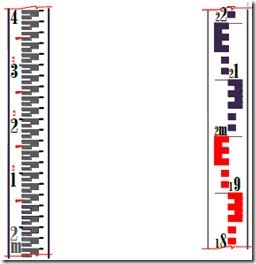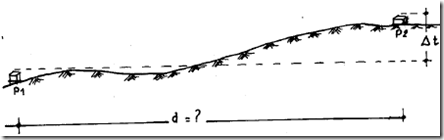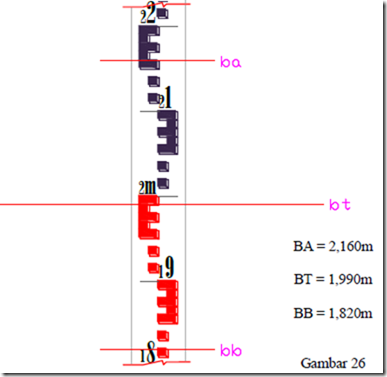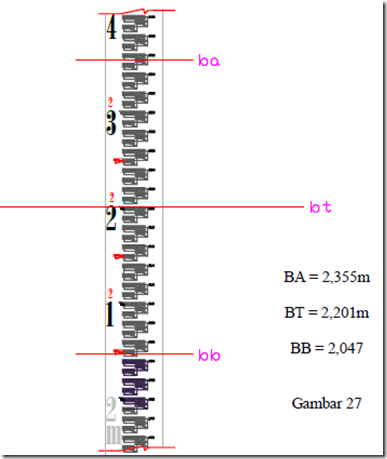Home > English > Reading Signs Measure

As described earlier, measuring buoy serves as a tool in determining the height difference by plane carpenter’s level, measuring signs usually consist of some kind, such as Figure 24 below.
Beacon beacon Interval Interval 5 mm 10 mmFigure 24

To get the height of a point, the necessary data from the measuring buoy readings, see sample results ukurr signs reading below:Figure 25

a. How  t (height difference)
b. How d (optical distance)
To accomplish the above, surveyors / surveyor should be able to carry out the reading of signs by plane. How to get a BA (yarn over), BT (thread center), BB (bottom thread) is as follows:From the example above, it can be concluded that in order to get the numbers in front of the comma, (0.000) should be based on the presence of the thread above (ba), the middle yarn (bt), thread under (b), in each column of 10 cm. See the example above, if ba = 0.875, meaning the value of the benchmark is a point in front of and behind the comma comma, 0.8. Signs reading of truth is indispensable in order to generate the right results the height difference and the distance is closer to the truth according to the data in the field.

Measurement of height difference there are three ways.
1. Measurement height difference when the aircraft above the point
2. Measurement height difference when the plane between two points
3. Measurement height difference when the air outside the 2 points"Tasikmalaya, Indonesia"